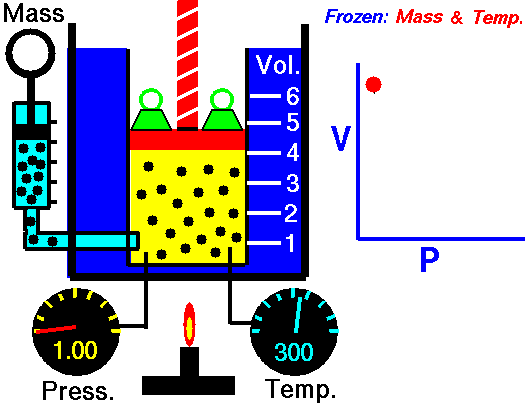## Boyle’s Law: Volume and Pressure

#### Learning Objective

• Apply Boyle’s Law using mathematical calculations.

#### Key Points

• According to Boyle’s Law, an inverse relationship exists between pressure and volume.
• Boyle’s Law holds true only if the number of molecules (n) and the temperature (T) are both constant.
• Boyle’s Law is used to predict the result of introducing a change in volume and pressure only, and only to the initial state of a fixed quantity of gas.
• The relationship for Boyle’s Law can be expressed as follows: P1V1 = P2V2, where P1 and V1 are the initial pressure and volume values, and P2 and V2 are the values of the pressure and volume of the gas after change.

#### Terms

• isothermin thermodynamics, a curve on a p-V diagram for an isothermal process
• Boyle’s lawthe absolute pressure and volume of a given mass of confined gas are inversely proportional, while the temperature remains unchanged within a closed system
• ideal gasa theoretical gas composed of a set of randomly-moving, non-interacting point particles

## Boyle’s Law

Boyle’s Law (sometimes referred to as the Boyle-Mariotte Law) states that the absolute pressure and volume of a given mass of confined gas are inversely proportional, provided the temperature remains unchanged within a closed system. This can be stated mathematically as follows:

[latex]P_1V_1=P_2V_2[/latex]

## History and Derivation of Boyle’s Law

The law was named after chemist and physicist Robert Boyle, who published the original law in 1662. Boyle showed that the volume of air trapped by a liquid in the closed short limb of a J-shaped tube decreased in exact proportion to the pressure produced by the liquid in the long part of the tube.Boyle’s LawAn animation of Boyle’s Law, showing the relationship between volume and pressure when mass and temperature are held constant.

The trapped air acted much like a spring, exerting a force opposing its compression. Boyle called this effect “the spring of the air” and published his results in a pamphlet with that title. The difference between the heights of the two mercury columns gives the pressure (76 cm = 1 atm), and the volume of the air is calculated from the length of the air column and the tubing diameter.

The law itself can be stated as follows: for a fixed amount of an ideal gas kept at a fixed temperature, P (pressure) and V (volume) are inversely proportional—that is, when one doubles, the other is reduced by half.

Remember that these relations hold true only if the number of molecules (n) and the temperature (T) are both constant.

## Example

In an industrial process, a gas confined to a volume of 1 L at a pressure of 20 atm is allowed to flow into a 12-L container by opening the valve that connects the two containers. What is the final pressure of the gas?

Set up the problem by setting up the known and unknown variables. In this case, the initial pressure is 20 atm (P1), the initial volume is 1 L (V1), and the new volume is 1L + 12 L = 13 L (V2), since the two containers are connected. The new pressure (P2) remains unknown.

P1V1 = P2V2

(20 atm)(1 L) = (P2)(13 L).

20 atom = (13) P2.

P2 = 1.54 atm.

The final pressure of the gas is 1.54 atm.